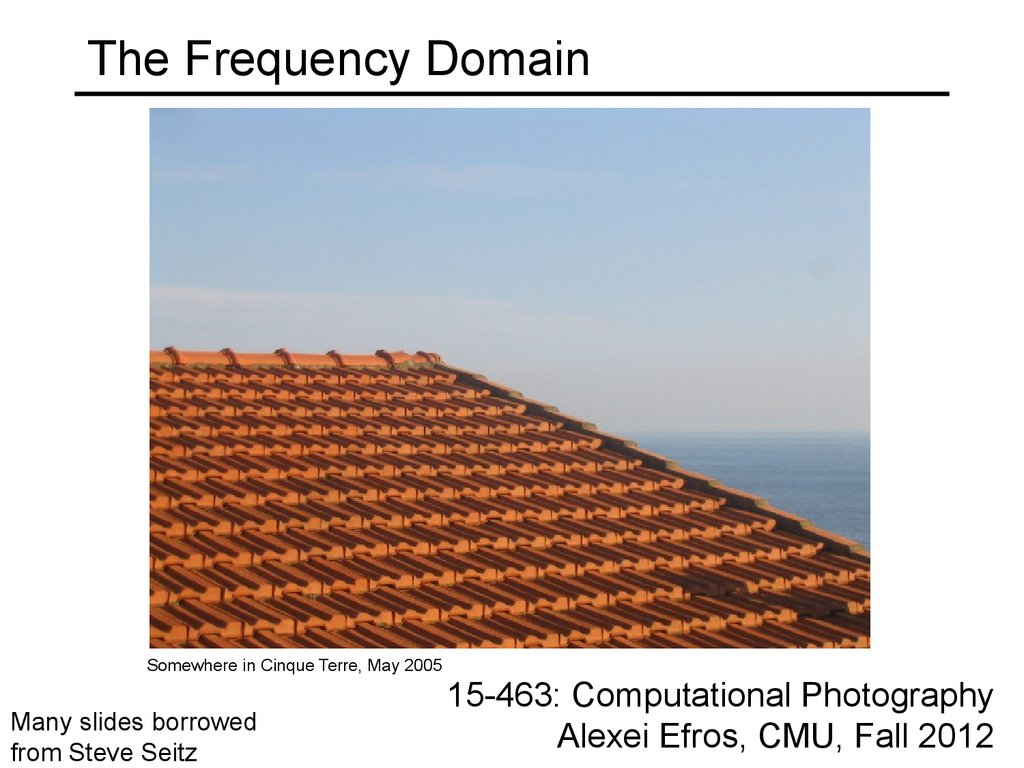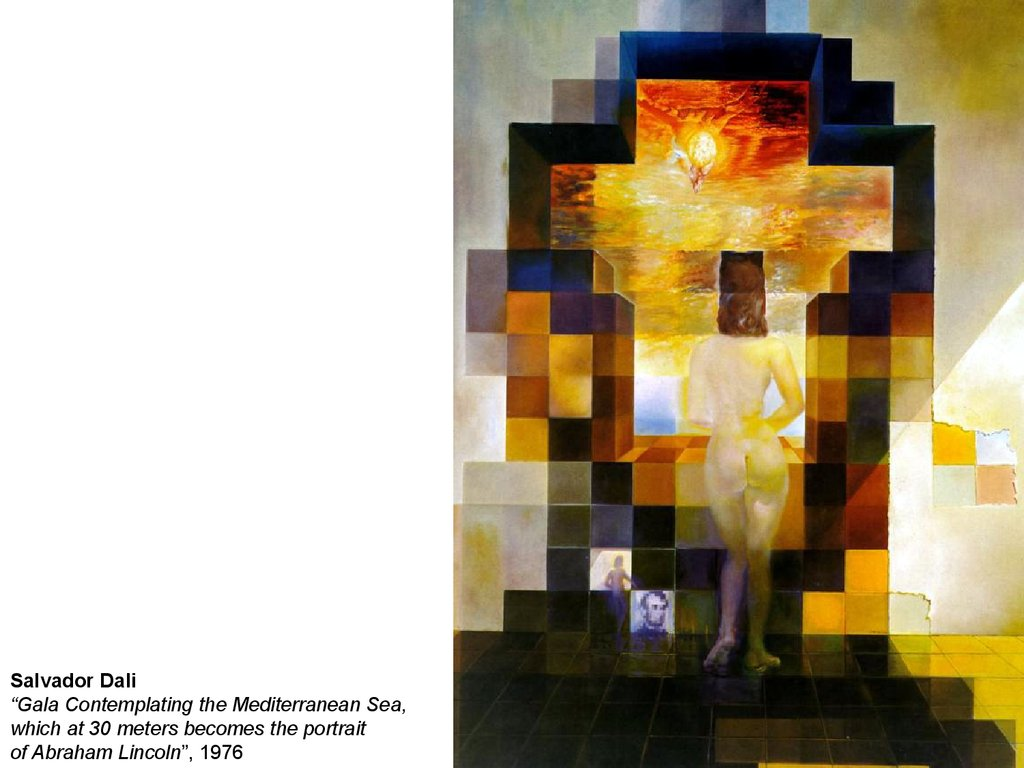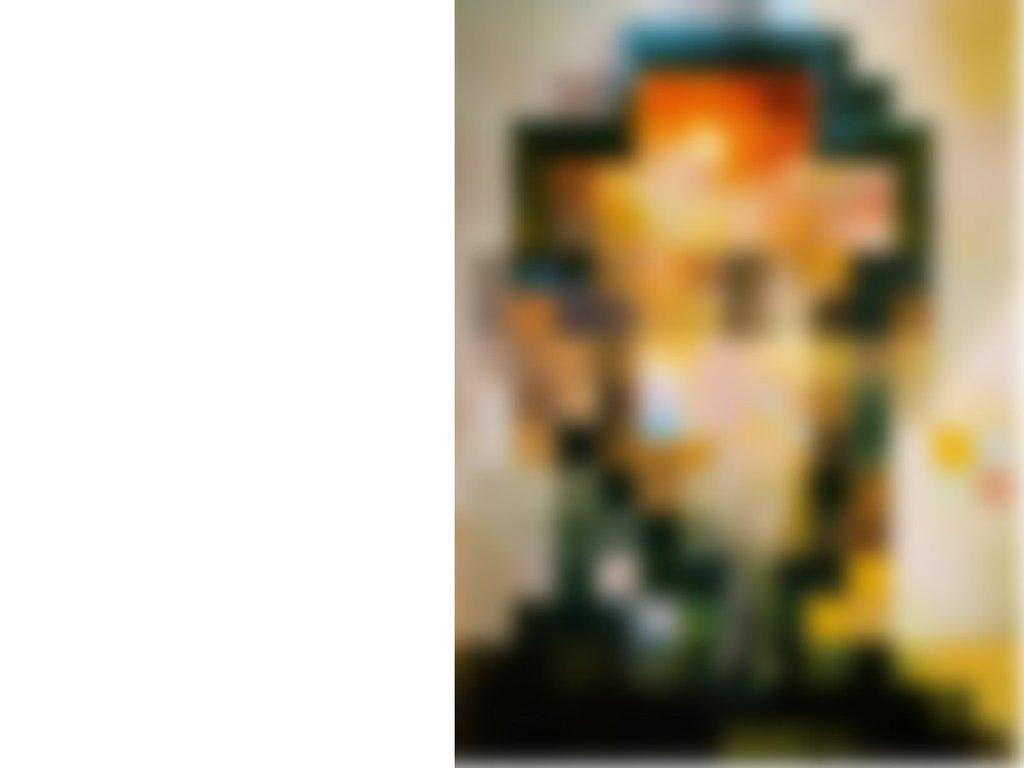# The Frequency Domain

## 1. The Frequency Domain

Somewhere in Cinque Terre, May 2005
Many slides borrowed
from Steve Seitz
15-463: Computational Photography
Alexei Efros, CMU, Fall 2012

## 2.

“Gala Contemplating the Mediterranean Sea,
which at 30 meters becomes the portrait
of Abraham Lincoln”, 1976

## 5. A nice set of basis

Teases away fast vs. slow changes in the image.
This change of basis has a special name…

## 6. Jean Baptiste Joseph Fourier (1768-1830)

...the manner in which the author arrives at
had crazy idea (1807): these equations is not exempt of difficulties
and...his analysis to integrate them still leaves
Any univariate function can
something to be desired on the score of
be rewritten as a weighted
generality and even rigour.
sum of sines and cosines of
different frequencies.
Don’t believe it?
• Neither did Lagrange,
Laplace, Poisson and
other big wigs
• Not translated into
English until 1878!
Laplace
But it’s (mostly) true!
• called Fourier Series
• there are some subtle
restrictions
Lagrange
Legendre

## 7. A sum of sines

Our building block:
Asin( x
Add enough of them to get
any signal f(x) you want!
How many degrees of
freedom?
What does each control?
Which one encodes the
coarse vs. fine structure of
the signal?

## 8. Fourier Transform

We want to understand the frequency of our signal. So,
let’s reparametrize the signal by instead of x:
f(x)
Fourier
Transform
F( )
For every from 0 to inf, F( ) holds the amplitude A
and phase of the corresponding sine Asin( x
• How can F hold both? Complex number trick!
F ( ) R( ) iI ( )
2
2
1 I ( )
A R( ) I ( )
tan
R( )
We can always go back:
F( )
Inverse Fourier
Transform
f(x)

## 9. Time and Frequency

example : g(t) = sin(2pf t) + (1/3)sin(2p(3f) t)

## 10. Time and Frequency

example : g(t) = sin(2pf t) + (1/3)sin(2p(3f) t)
=
+

## 11. Frequency Spectra

example : g(t) = sin(2pf t) + (1/3)sin(2p(3f) t)
=
+

## 12. Frequency Spectra

Usually, frequency is more interesting than the phase

=
=
+

=
=
+

=
=
+

=
=
+

=
=
+

¥
1
= Aå sin(2p kt )
k 1 k

M * f(x) = F( )
*
.
.
.
=

## 21. IFT: Just a change of basis

M-1 * F( ) = f(x)
*
.
.
.
=

## 23. Finally: Scary Math

i x
…not really scary: e cos( x ) i sin( x )
is hiding our old friend: Asin( x
phase can be encoded
by sin/cos pair
P cos( x ) Q sin( x ) A sin( x
Α P2 Q2
P
tan 1
Q
So it’s just our signal f(x) times sine at frequency

## 24. Extension to 2D

in Matlab, check out: imagesc(log(abs(fftshift(fft2(im)))));

## 25. Fourier analysis in images

Intensity Image
Fourier Image
http://sharp.bu.edu/~slehar/fourier/fourier.html#filtering

## 26. Signals can be composed

+
=
http://sharp.bu.edu/~slehar/fourier/fourier.html#filtering
More: http://www.cs.unm.edu/~brayer/vision/fourier.html

## 30. The Convolution Theorem

The greatest thing since sliced (banana) bread!
• The Fourier transform of the convolution of two
functions is the product of their Fourier transforms
F[ g h] F[ g ] F[h]
• The inverse Fourier transform of the product of two
Fourier transforms is the convolution of the two
inverse Fourier transforms
1
1
1
F [ gh] F [ g ] F [h]
• Convolution in spatial domain is equivalent to
multiplication in frequency domain!

|F(sx,sy)|
f(x,y)
*
h(x,y)
|H(sx,sy)|
g(x,y)
|G(sx,sy)|

## 32.

Filtering
Why does the Gaussian give a nice smooth
image, but the square filter give edgy
artifacts?
Gaussian
Box filter

Gaussian

Box Filter

## 36. Low-pass, Band-pass, High-pass filters

low-pass:
High-pass / band-pass:

original

## 39. What does blurring take away?

smoothed (5x5 Gaussian)

## 40. High-Pass filter

smoothed – original

## 41. Band-pass filtering

Gaussian Pyramid (low-pass images)
Laplacian Pyramid (subband images)
Created from Gaussian pyramid by subtraction

## 42. Laplacian Pyramid

Need this!
Original
image
How can we reconstruct (collapse) this
pyramid into the original image?

## 43. Why Laplacian?

Gaussian
delta function
Laplacian of Gaussian

## 44. Project 2: Hybrid Images

Gaussian Filter!
A. Oliva, A. Torralba, P.G. Schyns,
“Hybrid Images,” SIGGRAPH 2006
Laplacian Filter!
Project Instructions:
unit impulse
Gaussian Laplacian of Gaussian
http://www.cs.illinois.edu/class/fa10/cs498dwh/projects/hybrid/ComputationalPhotography_ProjectHybrid.html

## 45. Clues from Human Perception

Early processing in humans filters for various orientations and scales
of frequency
Perceptual cues in the mid frequencies dominate perception
When we see an image from far away, we are effectively subsampling
it
Early Visual Processing: Multi-scale edge and blob filters

## 46. Frequency Domain and Perception

Campbell-Robson contrast sensitivity curve

## 48.

Leonardo playing with peripheral vision

100
200
­
300
400
=
500
200
400

600
800
=

R
G
B

## 51. Lossy Image Compression (JPEG)

Block-based Discrete Cosine Transform (DCT)

## 52. Using DCT in JPEG

The first coefficient B(0,0) is the DC component,
the average intensity
The top-left coeffs represent low frequencies,
the bottom right – high frequencies

## 53. Image compression using DCT

Quantize
• More coarsely for high frequencies (which also tend to have smaller
values)
• Many quantized high frequency values will be zero
Encode
• Can decode with inverse dct
Filter responses
Quantization table
Quantized values

## 54. JPEG Compression Summary

Subsample color by factor of 2
People have bad resolution for color
Split into blocks (8x8, typically), subtract 128
For each block
a. Compute DCT coefficients for
b. Coarsely quantize

Many high frequency components will become zero
c. Encode (e.g., with Huffman coding)
http://en.wikipedia.org/wiki/YCbCr
http://en.wikipedia.org/wiki/JPEG

## 55. Block size in JPEG

Block size
• small block
– faster
– correlation exists between neighboring pixels
• large block
– better compression in smooth regions
• It’s 8x8 in standard JPEG

## 56. JPEG compression comparison

89k
12k

The gradient points in the direction of most rapid change in intensity
The gradient direction is given by:
• how does this relate to the direction of the edge?
The edge strength is given by the gradient magnitude

## 58. Effects of noise

Consider a single row or column of the image
• Plotting intensity as a function of position gives a signal
How to compute a derivative?
Where is the edge?

## 59. Solution: smooth first

Where is the edge? Look for peaks in

## 60. Derivative theorem of convolution

This saves us one operation:

## 61. Laplacian of Gaussian

Consider
Laplacian of Gaussian
operator
Where is the edge?
Zero-crossings of bottom graph

## 62. 2D edge detection filters

Laplacian of Gaussian
Gaussian
derivative of Gaussian
is the Laplacian operator:

## 63. Try this in MATLAB

g = fspecial('gaussian',15,2);
imagesc(g);  colormap(gray);
surfl(g)
gclown = conv2(clown,g,'same');
imagesc(conv2(clown,[­1 1],'same'));
imagesc(conv2(gclown,[­1 1],'same'));
dx = conv2(g,[­1 1],'same');
imagesc(conv2(clown,dx,'same'));
lg = fspecial('log',15,2);
lclown = conv2(clown,lg,'same');
imagesc(lclown)
imagesc(clown + .2*lclown)